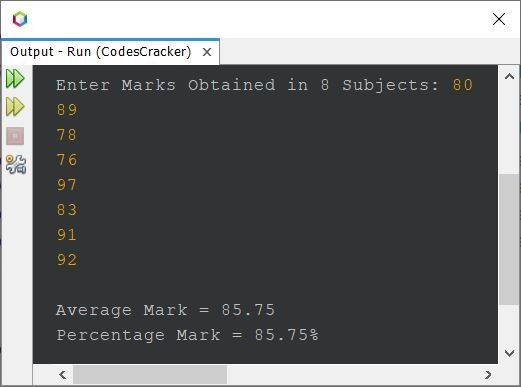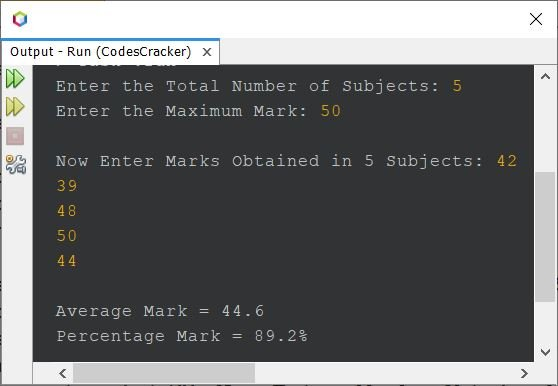# Java Program to Calculate Average and Percentage Marks

This article is created to cover a program in Java that find and prints the average and percentage mark, based on the marks obtained in some number of subjects, entered by user at run-time of the program.

## Find Average & Percentage Mark in Java - Basic Version

The question is, write a Java program to find average and percentage marks based on marks received in 8 subjects. The program given below is its answer:

```import java.util.Scanner;

public class CodesCracker
{
public static void main(String[] args)
{
float[] marks = new float;
float sum=0, avg, perc;
int i;
Scanner scan = new Scanner(System.in);

System.out.print("Enter Marks Obtained in 8 Subjects: ");
for(i=0; i<8; i++)
marks[i] = scan.nextFloat();

for(i=0; i<8; i++)
sum = sum + marks[i];

avg = sum/8;
perc = avg;
System.out.println("\nAverage Mark = " +avg);
System.out.println("Percentage Mark = " +perc+ "%");
}
}```

The snapshot given below shows the sample run of above Java program with user input 80, 89, 78, 76, 97, 83, 91, 92 as marks obtained in 8 subjects:Since the program given below is based on the maximum mark of 100. Therefore, you're seeing the output of average and percentage, as same.

## Find Average & Percentage Mark in Java - Complete Version

Since previous program is based on 8 subjects only. Therefore I've modified that program to allow user define the number of subject too along with marks obtained in each subjects. The maximum mark is also allowed to define, by user at run-time of the program.

```import java.util.Scanner;

public class CodesCracker
{
public static void main(String[] args)
{
float[] marks = new float;
float sum=0, avg, perc;
Scanner scan = new Scanner(System.in);

System.out.print("Enter the Total Number of Subjects: ");
int tot = scan.nextInt();
System.out.print("Enter the Maximum Mark: ");
int max = scan.nextInt();
System.out.print("\nNow Enter Marks Obtained in " +tot+" Subjects: ");
for(int i=0; i<tot; i++)
{
marks[i] = scan.nextFloat();
sum += marks[i];
}

avg = sum/tot;
perc = (sum*100)/(tot*max);
System.out.println("\nAverage Mark = " +avg);
System.out.println("Percentage Mark = " +perc+ "%");
}
}```

Here is its sample run with user inputs, 5 as number of subjects, 50 as maximum marks, 42, 39, 48, 50, 44 as five subject marks:Now see the output of average and percentage. That is, both are different, because this time, the maximum mark is 50. The percentage always tells the average marks out of 100. Where as the average just prints the sum of total subject divided by the total number of subjects.

#### Same Program in Other Languages

Java Online Test

« Previous Program Next Program »